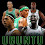## Monday, March 24, 2014

### 33 Watch: Larry Bird, the Boston Celtics and the NBA

Not only did Larry Bird wear the number 33 for the Boston Celtics during his playing days, he also has a last name of Bird with "33" numerology and played for a team located in one of three states with "33 numerology".  Let us examine.
• Bird = 2+9+18+4 = 33
• Larry = 12+1+18+18+25 = 74, reduces to 11
Interestingly enough, Jesus, who was crucified at the age of 33 after performing 33 miracles, also has "74" numerology.
• Jesus = 10+5+19+21+19 = 74
• Cross = 3+18+15+19+19 = 74
Again, Boston is located in Massachusetts.
• Boston = 2+6+1+2+6+5 = 22
• Celtics = 2+5+3+2+9+3+1 = 25
• Boston Celtics =47
• 47 = Inverse of 74, both reduce to 11
• Massachusetts = 4+1+1+1+1+3+8+3+1+5+2+2+1 = 33
Larry Bird was listed as being 6'9" and 220 lbs.  His birthday is recorded as December 7, 1956, a date with 31 numerology.
• 12/31/1956 = 1+2+7+1+9+5+6 = 31
In total, Larry Bird has some key numbers in numerology that please the powers that be, and make him a perfect match with stars such as Magic, Michael, Kobe, and LeBron.  If you doubt the NBA is a "33 League", please read the other NBA posts on this blog.  The evidence is overwhelming.
• Magic = 13+1+7+9+3 = 33
• Michael = 4+9+3+8+1+5+3 =33
• Kobe = 11+15+2+5 = 33
• Vincent = 4+9+5+3+5+5+2 = 33
• Riley = 9+9+3+5+7 = 33
• LeBron = 12+5+2+18+15+14 = 66
LeBron James was drafted by the Cleveland Cavaliers.
• Cleveland = 3+3+5+4+5+3+1+5+4 = 33
While the talent in the NBA is real, so is the rigging.  In Larry Bird's 13-year playing career, he was an All-Star 12 times and league MVP 3 times.  To date, Larry Bird is the only person in NBA history to win the MVP, Coach of the Year and Executive of the Year awards, which he has accomplished the latter two while with the Indiana Pacers.  Larry Bird is also a three-time NBA champion, winning in 1981, 1984 and 1986.  His number, 33, has been retired by the Boston Celtics.  What I wonder, is how many to date, have caught the fact that the last name Bird also equates to 33?

In Bird's playing career, his two greatest teammates were said to be Robert Parrish and Kevin McHale.  In other words, there were 3 main stars behind the Celtics dominance of the early and mid 80s.
• Robert = 9+6+2+5+9+2 = 33
• Parrish = 7+1+9+9+9+1+8 = 44
• Robert Parish = 33+44 = 77
So, Robert Parrish has master numerology as well, and before we examine Kevin McHale's name, let us realize that 7x7 (from the 77 in Robert Parish's name) = 49, and that 77 and 49 are also extremely important numbers to the powers that be; just like master numbers 11, 22, 33, etc.
• Kevin = 2+5+4+9+5 = 25
• McHale = 4+3+8+1+3+5 = 24
• Kevin McHale = 49
The Boston Celtics have only won one championship since their dominance in the 1980s.  That championship team was lead by the trio of Kevin Garnett, Ray Allen and Paul Pierce.  For a moment, let's focus on Ray Allen, who truly has a first name of Walter, and a middle name of Ray, thus 'Walter Ray Allen'.
• Ray = 18+1+25 = 44
• Allen = 1+12+12+5+14 = 44
• Walter = 5+1+3+2+5+9 = 24
• Walter Ray Allen = 25+44+44 = 113 (11x3 = 33)
Ray Allen also has something else in common with Larry Bird, the numerology of his birthday.  Ray Allen was born July 20, 1975.
• 7/20/1975 = 7+2+1+9+7+5 = 31
Thirty-one to thirty-three are the ranging degrees of Freemasonry.  Also, whereas Larry has a first name matching the numerology of Jesus (from above), Ray Allen once starred in a movie opposite of Denzel Washington, called He Got Game, where his character was named "Jesus Shuttlesworth".  Perhaps it isn't all a coincidence.  Perhaps there is something we have never been told about the NBA and what is really going on with their players behind the scenes.  Looking at the facts, it seems that players names and NBA success aren't arbitrary.

Stay tuned, and please read the posts about Michael, Kobe, Pat Riley, Magic and more than can be found on this blog.  Until then, think about this.  Is it possible the Illuminati has a basketball gym somewhere, and they're raising children with the right genetics to be stars in their owned and controlled leagues, such as the NBA?  Anyone who has ever seen Ray Allen shoot the ball knows he has one of the prettiest strokes there could ever be... it makes one wonder where he learned it, and how much time he dedicated to the craft coming up?  Maybe Larry Bird knows.

1.This is superb.

2.Thanks KWAPT. I see your a Celtics fan, so glad you can appreciate it.

3.Again, Boston is located in Massachusetts.
Boston = 2+6+1+2+6+5 = 22
How?.